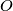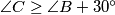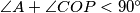### IMO Shortlist 2001 problem G2

Kvaliteta:
Avg: 0.0
Težina:
Avg: 6.0
Consider an acute-angled triangle$ABC$. Let$P$ be the foot of the altitude of triangle$ABC$ issuing from the vertex$A$, and let$O$ be the circumcenter of triangle$ABC$. Assume that$\angle C \geq \angle B+30^{\circ}$. Prove that$\angle A+\angle COP < 90^{\circ}$.
Source: Međunarodna matematička olimpijada, shortlist 2001